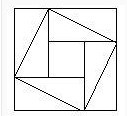# 空心方阵问题公式

（外边每边人数-层数）×层数---表示的是弦图中的一个长方形
×4---4个长方形---------------------------------------------------------------------------------------------------------

A1=4X-4

A2=4(X-2)-4

A3=4(X-4)-4
A4=4(X-6)-4

SN=NA1+[N(N-1)D]/2

SN=N(4X-4)-4(N^2-N)
SN=4N(X-N)

>>点击查看空心方阵公式专题，阅读更多相关文章！